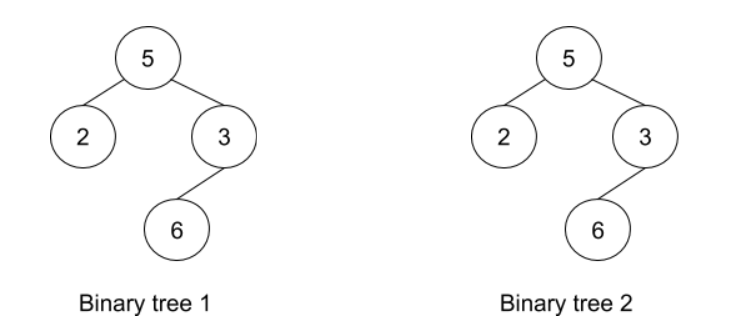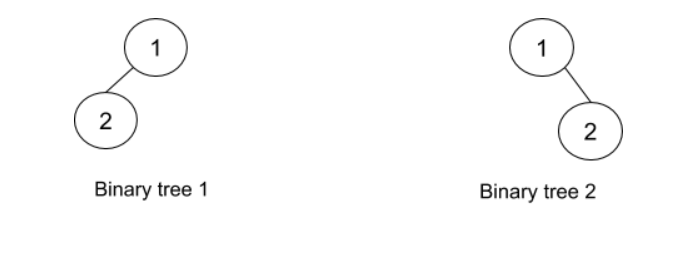New update is available. Click here to update.Close
Topic list
Check Identical Trees
MEDIUM
20 mins22 upvotes
Other topics
Problem solved
Skill meterStrings
-
-Matrices (2D Arrays)
-
--
-Sorting
-
-Binary Search
-
-Stacks & Queues
-
-Trees
-
-Graph
-
-Dynamic Programming
-
-Greedy
-
-Tries
-
-Arrays
-
-SQL
-
-Binary Search Trees
-
-Heap
-
-Bit Manipulation
-
-
Solve problems & track your progress
Checkout your overall progress in every topic here
BecomeSensei
in DSA topics
Open the topic and solve more problems associated with it to improve your skills
Check out the skill meter for every topic
See how many problems you are left with to solve for cracking any stage. Score more than zero to get your progress counted.

# Check Identical Trees

Medium0/80
Avg time to solve 20 mins
Success Rate 85 %Share22 upvotes

## Problem Statement

#### Below is the example and explanation of Identical trees.

##### Example :
`````` For the trees given below:-
````````````The given trees are identical as:-
1. The number of nodes in both trees is the same.
2. The number of edges in both trees is the same.
3. The data for root for both the trees is the same i.e 5.
4. The data of root -> left (root’s left child) for both the trees is the same i.e 2.
5. The data of root -> right (root’s right child) for both the trees is the same i.e 3.
6. The data of root -> right -> left ( left child of root’s right child) for both the trees is the same i.e 6.
7. Nodes with data 2 and 6 are the leaf nodes for both the binary trees.
``````
Detailed explanation ( Input/output format, Notes, Constraints, Images )##### Sample input 1 :
``````2
5 2 3 -1 6 -1 -1 -1 -1
5 2 3 -1 6 -1 -1 -1 -1
1 -1 2 -1 -1
1 2 -1 -1 -1
``````
##### Sample output 1 :
``````True
False
``````
##### Explanation for Sample Input 1 :
``````For test case 1: Refer to the example given above in the problem description.

For test case2: The trees given in the input can be represented as below:-
````````````Although the following conditions satisfy:

The number of nodes in both trees is the same.
The number of edges in both trees is the same.
The data for root for both the trees is the same i.e 1.

It’s visible from the pictorial representation that the trees are not identical. Reason being:
In Binary tree 1, root’s right is NULL but it’s not true for Binary tree 2.
In Binary tree 1, root’s left is not NULL but it’s not true for Binary tree 2.
``````
##### Sample input 2 :
``````2
4 9 -1 1 2 -1 -1 -1 -1
4 9 -1 1 2 -1 -1 -1 -1
6 2 3 4 5 7 8 -1 -1 -1 -1 -1 -1 -1 -1
6 2 3 4 5 8 7 -1 -1 -1 -1 -1 -1 -1 -1
``````
##### Sample output 2 :
``````True
False
``````AutoConsole